Rectangular Prisms
Triangular Prisms
SA of Cylinders
Cylinders, Cones & Spheres
All o' them!
100

Find the formula for the lateral surface area of a rectangular prism.

What is S = Ph?

100

Find the formula for lateral surface area of a triangular prism.

What is S = Ph?

100

Find the formula for lateral surface area of a cylinder.

What is S = 2pirh?

100

Find the formula for the volume of a cylinder.

What is V = pir^2h?

100

Find the formula for the volume of a sphere.

What is V = 4/3pir^3?

200

Find the formula for total surface area of a rectangular prism.

What is S = Ph + 2B?

200

Find the formula for total surface area of a triangular prism.

What is S = Ph + 2B?

200

Find the formula for total surface area of a cylinder.

What is S = 2pirh + 2pir2?

200

Find the formula for the volume of a cone.

What is V = 1/3pir^2h?

200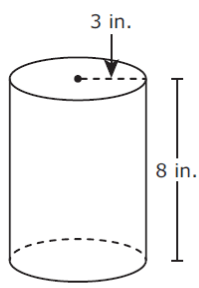Find the lateral surface area.

What is 150.8 in2?

300Find the total surface area.

What is 102.16 in2?

300Find the lateral surface area with 15cm as the height of the prism.

What is 270cm2?

300Find the lateral surface area. (Round to the nearest tenth)

What is 282.7 in2?

300

Double Jeopardy!!!!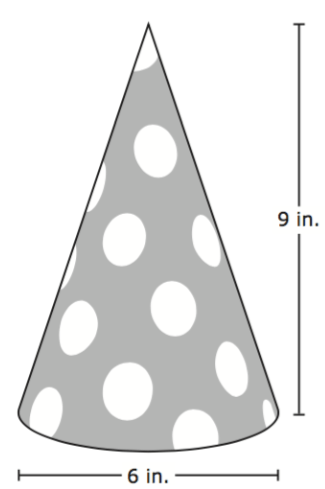Find the volume of the cone.

What is 84.8 in3?
300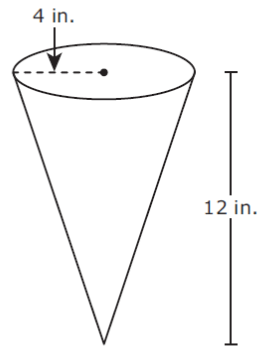Find the volume.

What is 201.1 in3?

400

Double Jeopardy!!!!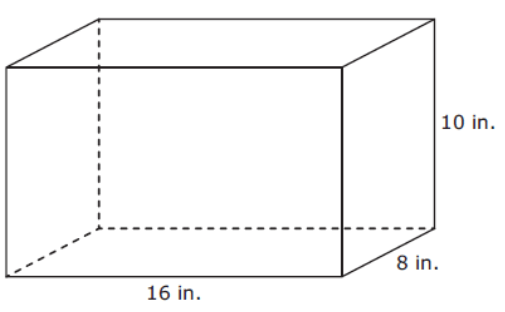Find the lateral surface area.

What is 480 in2?

400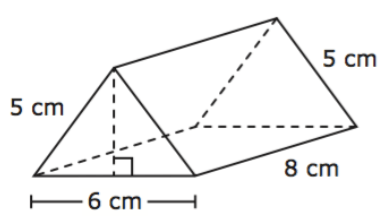Find the lateral surface area.

What is 128 cm2?

400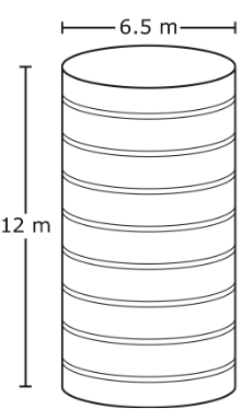Find the amount of wrapping paper needed to give this as a gift.

What is 311.4 m2?

400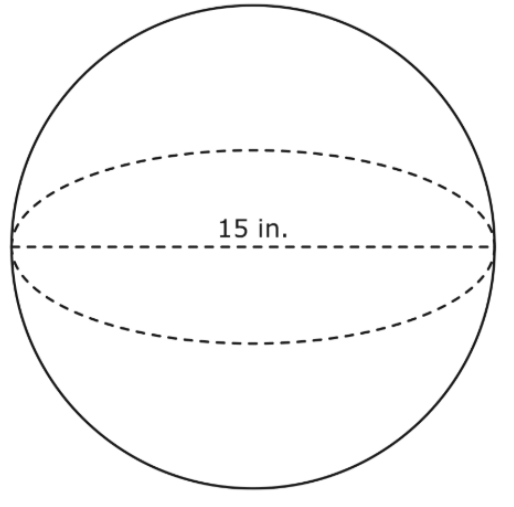Find the volume of the sphere.

What is 1767.1 in3?

400Find the total surface area.

What is 736 in2?

500
Susana has box of cards she wants to wrap as a gift. If the box is 0.5in long, 1in wide, and 2in tall, find the surface area needed to wrap the box of cards.

What is 7 in2?

500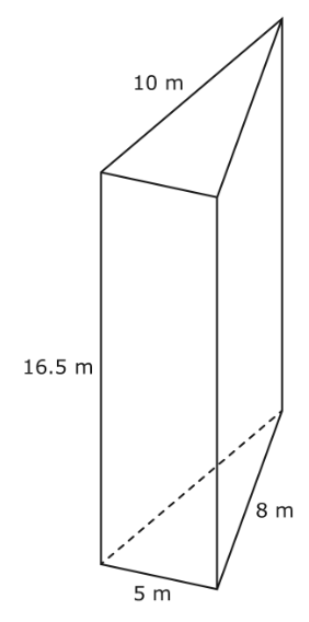Find the lateral surface area.

What is 379.5 m2?

500Find the total surface area.

What is 339.3 in2?

500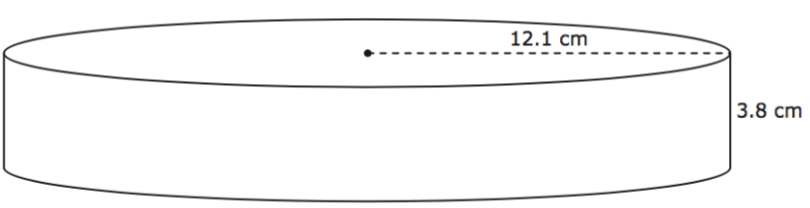Find the volume of the shape above.

What is 1747.9 cm3?

500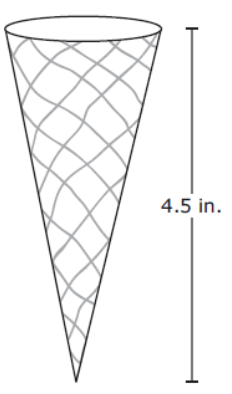Find the volume of a cylinder if the diameter is 14in and the height is the same as the cone above.

What is 692.7 in3?

1000

Final Jeopardy:

John wants to sample paint rollers to see which covers a greater amount of area. He samples one roller that has a diameter of 5.75cm and a length of 10cm. Find the amount of surface painted with one complete rotation of the paint roller.

What is 180.6 cm2?

1000

Final Jeopardy:

Joe has a can and an ice cream cone. The can has a height of 10.84in and a diameter of 10.84in. If the cone has the same measurements as the can, find the volume of the cone.

What is 333.5 in3?

1000

Final Jeopardy:

If a cylinder, a sphere, and a cone all have a radius of 5 and a height of 10, find the shape that has the most volume.

What is a cylinder?

1000

Final Jeopardy:

A cylinder, a cone, and a sphere all have the same measurements. If the sphere has a radius of 10 and the cone has a height of 20, find the diameter of the cylinder.

What is 20?

1000

Final Jeopardy:

Jay wants to wrap his Funko Pop as a birthday gift for his friend. The box measures 5in x 7in x 5in (L x W x H). Find the amount of surface area Jay will need to cover with the wrapping paper.

What is 190 in2?

Click to zoom